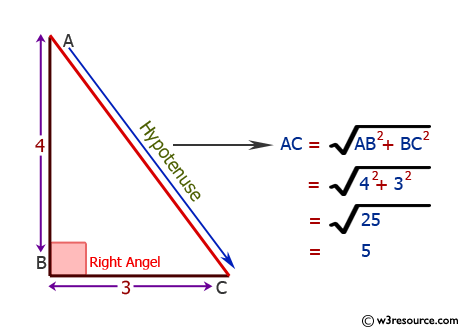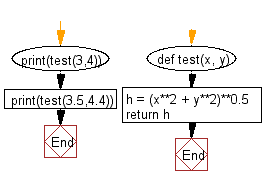﻿ Python: Calculate the hypotenuse of a right angled triangle - w3resource# Python: Calculate the hypotenuse of a right angled triangle

## Python Basic: Exercise-60 with Solution

Write a Python program to calculate the hypotenuse of a right angled triangle.

From Wikipedia,
A right triangle or right-angled triangle, or more formally an orthogonal triangle, is a triangle in which one angle is a right angle. The relation between the sides and angles of a right triangle is the basis for trigonometry. The side opposite the right angle is called the hypotenuse. If the lengths of all three sides of a right triangle are integers, the triangle is said to be a Pythagorean triangle and its side lengths are collectively known as a Pythagorean triple.

Pictorial Presentation:Sample Solution-1:

Python Code :

``````from math import sqrt
print("Input lengths of shorter triangle sides:")
a = float(input("a: "))
b = float(input("b: "))
c = sqrt(a**2 + b**2)
print("The length of the hypotenuse is:", c )
```
```

Sample Output:

```Input lengths of shorter triangle sides:
a:  3
b:  4
The length of the hypotenuse is: 5.0
```

## Visualize Python code execution:

The following tool visualize what the computer is doing step-by-step as it executes the said program:

Sample Solution-2:

Python Code:

``````def test(x, y):
h = (x**2 + y**2)**0.5
return h
print(test(3,4))
print(test(3.5,4.4))
```
```

Sample Output:

```5.0
5.622277118748239
```

Flowchart:## Visualize Python code execution:

The following tool visualize what the computer is doing step-by-step as it executes the said program:

Python Code Editor:

Have another way to solve this solution? Contribute your code (and comments) through Disqus.

What is the difficulty level of this exercise?

Test your Python skills with w3resource's quiz

﻿

## Python: Tips of the Day

Try-catch-else construct:

```try:
foo()
except Exception:
print("Exception occured")
else:
print("Exception didnt occur")
finally:
print("Always gets here")
```